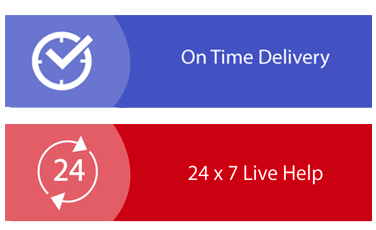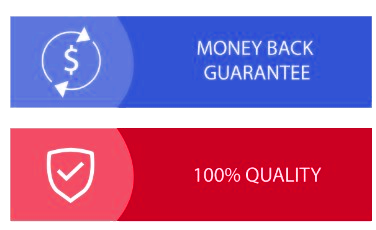× Limited Time Offer ! FLAT 30-40% off - Grab Deal Before It’s Gone. Order Now

## ITSU2011 Computational Mathematics Assignment Sample

### Question

Q1) 16 teams enter a competition. They are divided up into four Pools (A, B, C and D) of four teams each.

Every team plays one match against the other teams in its Pool. After the Pool matches are completed:

- the winner of Pool A plays the second placed team of Pool B
- the winner of Pool B plays the second placed team of Pool A
- the winner of Pool C plays the second placed team of Pool D
- the winner of Pool D plays the second placed team of Pool C

The winners of these four matches then play semi-finals, and the winners of the semi-finals play in the final.

How many matches are played altogether?
[5 marks]

Q2) A restaurant offers 5 choices of appetizer, 10 choices of main meal and 4 choices of dessert. A customer can choose to eat just one course, or two different courses, or all three courses. Assuming all choices are available, how many different possible meals does the restaurant offer?
[5 marks]

Q3) Find the number of spanning trees in the following graph.
[5 marks]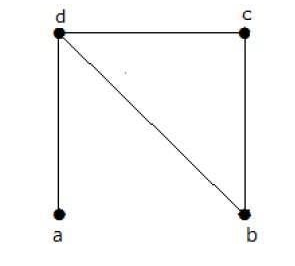Q4) Suppose a delivery person needs to deliver packages to three locations and return to the home office A. Using the graph shown below, find the shortest route if the weights on the graph represent distance in kilometers.
[5 marks]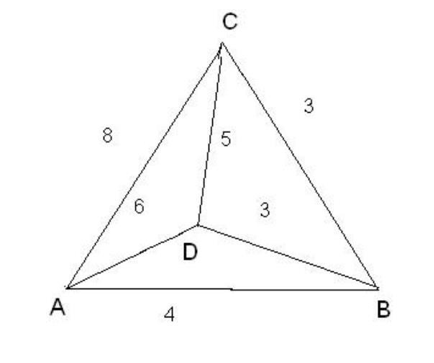## Solution

### Question 1

As per given data, there are four Pools named as A, B, C and D of four teams each for assignment help
Let us assume that Pool A has team 1, 2, 3, and 4 respectively.
Number of games in Pool A = (4 – 1)! = 3! = 3 * 2 = 6
So, total number of games of all four Pools = 4 * 6 = 24
Now, as per given instructions,
Number of quarter finals = 4
Number of semi-finals = 2
And, number of finals = 1
Therefore, total number of matches played together = 24 + 4 + 2 + 1 = 31

### Question 2

As per given data, the summary of choices are:
Number of choices of appetizer = 5
Number of choices of appetizer = 10
Number of choices of appetizer = 4
If customer eats only 1 meal, then
Number of ways = 5 + 10 + 4 = 19
If customer has 2 course meal, then
Number of ways = 5 * 10 + 5 * 4 + 10 * 4 = 50 + 20 + 40 = 110
If customer has 3 course meal, then
Number of ways = 5 * 10 * 4 = 200
Therefore, total number of possible meals = 19 + 110 + 200 = 329

### Question 3

The given graph is as follows: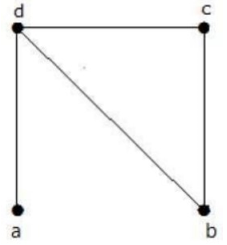For the above graph, the Laplacian matrix can be written as: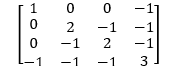Since from graph theory, we know that number of spanning trees is equal to the co-factor of Laplacian matrix.

So,

Co-factor of the above matrix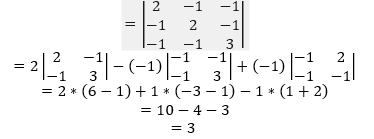Therefore, number of spanning trees is 3.

### Question 4

The provided graph is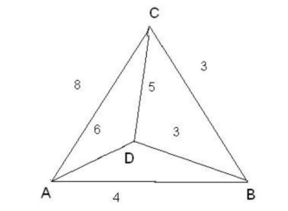Let the delivery person start from the home office A.

From the graph, it is clear that three edges AB, AD and AC are belongs to vertex A having weightage 4, 6 and 8 respectively.

Since, minimum weight of the edge from A is 4 (i.e. AB). So, the person goes to B from A.

In the similar way, the edge BD and BC have the minimum weight as 3. So, the person goes to C from B.

Similarly from C, edge CD have the minimum weight as 5. So, the person goes to D from C and then goes to home office A.

Therefore, the shortest route is

A – B – C – D – A with the weights 4 + 3 + 5 + 6 = 18
Hence, the optimal solution is 18 and path is ABCDA.

Fill the form to continue reading